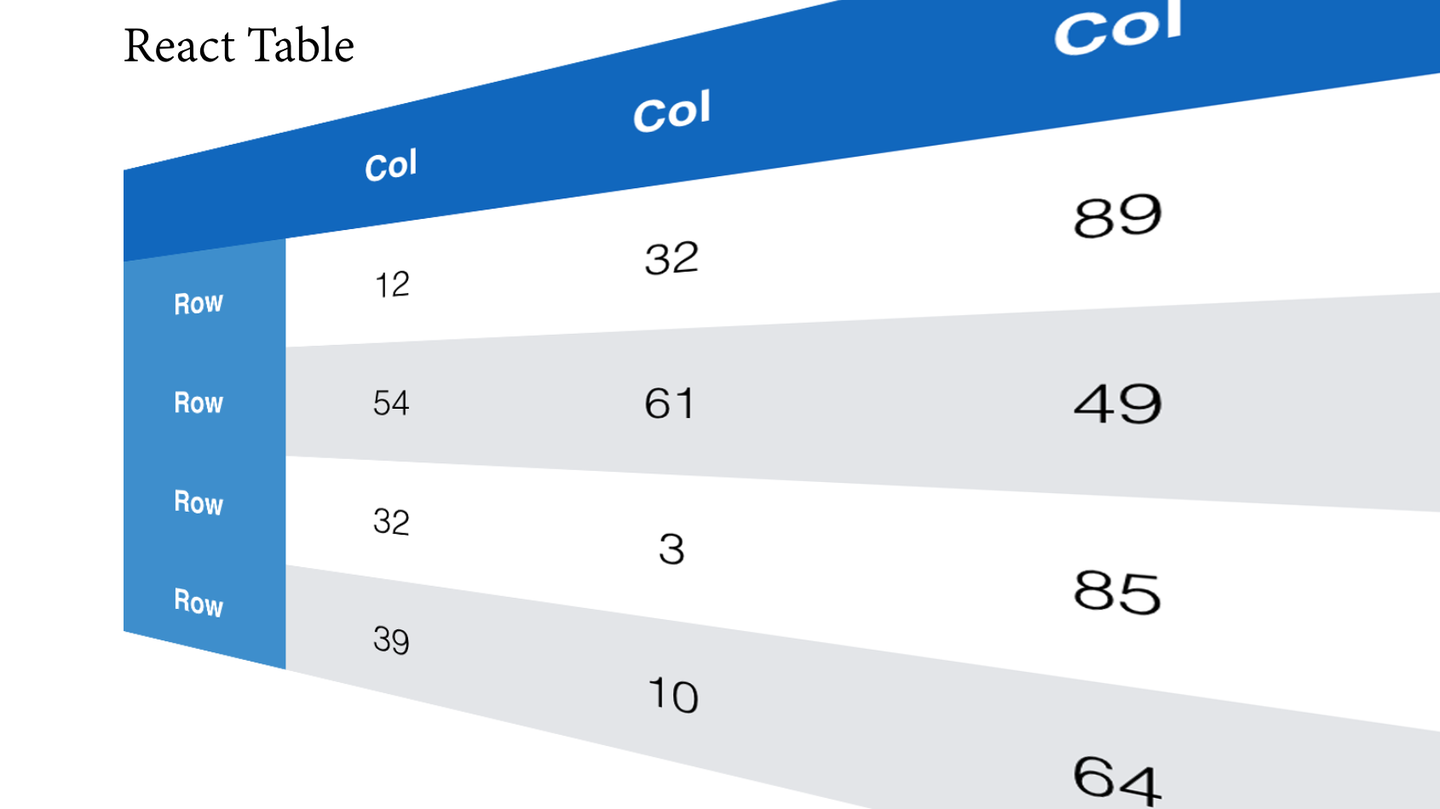Table 是最常用展示数据的方式之一，可是一个产品中往往很多非常类似的 Table，但是我们碰到的情况往往是 Table A 要排序，Table B 不需要排序，等等这种看起来非常类似，但是又不完全相同的表格。这种情况下，到底要不要抽取一个公共的 Table 组件呢？对于这个问题，我们团队也纠结了很久，先后开发了多个版本的 Table 组件，在最近的一个项目中，产出了第三版 Table 组件，能够较好的解决灵活性和公共逻辑抽取的问题。本文将会详细的讲述这种 Table 组件解决方案产出的过程和一些思考。

## Table 的常见实现

``````import React, { Component } from 'react';

const columnOpts = [
{ key: 'a', name: 'col-a' },
{ key: 'b', name: 'col-b' },
];

function SomeTable(props) {
const { data } = props;

return (
<div className="some-table">
{
columnOpts.map((opt, colIndex) => (
<li key={`col-\${colIndex}`}>{opt.name}</li>
))
}
</ul>
<ul className="table-body">
{
data.map((entry, rowIndex) => (
<li key={`row-\${rowIndex}`}>
{
columnOpts.map((opt, colIndex) => (
<span key={`col-\${colIndex}`}>{entry[opt.key]}</span>
))
}
</li>
))
}
</ul>
</div>
);
}
``````

• 每次写表格需要写很多布局类的样式

• 重复代码很多，而且项目成员之间很难达到统一，A 可能喜欢用表格来布局，B 可能喜欢用 ul 来布局

• 相似但是不完全相同的表格很难复用

## 抽象过程

• 输入数据源较统一，一般为对象数组

• tbody 中的部分单元格只是简单的读取一些值，很多单元格的都有自己的逻辑，但是在一个产品中通常很多类似的单元格

• 列是有顺序的，更适合以列为单位来添加布局样式

• 接收一个 对象数组所有列的配置 为参数，自动创建基础的表格内容

``````const columnOpts =  [
{ key: 'a', name: 'col-a', onRenderTd: () => {} },
{ key: 'b', name: 'col-b', onRenderTh: () => {}, onRenderTd: () => {} },
];

<Table data={data} columnOpts={columnOpts} />
``````

``````<Table data={data}>
<Column dataKey="a" name="col-a" td={onRenderTd} />
<Column dataKey="b" name="col-b" td={onRenderTd} th={onRenderTh} />
</Table>
``````

### 优化

``````import React, { PropTypes, Component } from 'react';

const propTypes = {
name: PropTypes.string,
dataKey: PropTypes.string.isRequired,
align: PropTypes.oneOf(['left', 'center', 'right']),
width: PropTypes.oneOfType([PropTypes.number, PropTypes.string]),
th: PropTypes.oneOfType([PropTypes.element, PropTypes.func]),
td: PropTypes.oneOfType([
PropTypes.element, PropTypes.func, PropTypes.oneOf([
'int', 'float', 'percent', 'changeRate'
])
]),
};

const defaultProps = {
align: 'left',
};

function Column() {
return null;
}

Column.propTypes = propTypes;
Column.defaultProps = defaultProps;

export default Column;
``````

td 的类型就更复杂了，不仅能够接收 function 和 ReactElement 这两种类型，还有 int, float, percent, changeRate 这三种类型是最常用的数据类型，这样方便我们可以在 Table 里面根据类型对数据做格式化，省去了项目成员中很多重复的代码。

``````const getDisplayName = (el) => {
return el && el.type && (el.type.displayName || el.type.name);
};

const renderChangeRate = (changeRate) => { ... };

const renderThs = (columns) => {
return columns.map((col, index) => {
const { name, dataKey, th } = col.props;
const props = { name, dataKey, colIndex: index };
let content;
let className;

if (React.isValidElement(th)) {
content = React.cloneElement(th, props);
className = getDisplayName(th);
} else if (_.isFunction(th)) {
content = th(props);
} else {
content = name || '';
}

return (
<th
key={`th-\${index}`}
style={getStyle(col.props)}
className={`table-th col-\${index} col-\${dataKey} \${className || ''}`}
>
{content}
</th>
);
});
};

const renderTds = (data, entry, columns, rowIndex) => {
return columns.map((col, index) => {
const { dataKey, td } = col.props;
const value = getValueOfTd(entry, dataKey);
const props = { data, rowData: entry, tdValue: value, dataKey, rowIndex, colIndex: index };

let content;
let className;
if (React.isValidElement(td)) {
content = React.cloneElement(td, props);
className = getDisplayName(td);
} else if (td === 'changeRate') {
content = renderChangeRate(value || '');
} else if (_.isFunction(td)) {
content = td(props);
} else {
content = formatIndex(parseValueOfTd(value), dataKey, td);
}

return (
<td
key={`td-\${index}`}
style={getStyle(col.props)}
className={`table-td col-\${index} col-\${dataKey} \${className || ''}`}
>
{content}
</td>
);
});
};

const renderRows = (data, columns) => {
if (!data || !data.length) {return null;}

return data.map((entry, index) => {
return (
<tr className="table-tbody-tr" key={`tr-\${index}`}>
{renderTds(data, entry, columns, index)}
</tr>
);
});
};

function Table(props) {
const { children, data, className } = props;
const columns = findChildrenByType(children, Column);

return (
<div className={`table-container \${className || ''}`}>
<table className="base-table">
{hasNames(columns) && (
{renderThs(columns)}
</tr>
)}
<tbody>{renderRows(data, columns)}</tbody>
</table>
</div>
);
}
``````

## 单元格示例

``````class SortableTh extends Component {
static displayName = 'SortableTh';

static propTypes = {
...,
initialOrder: PropTypes.oneOf(['asc', 'desc']),
order: PropTypes.oneOf(['asc', 'desc', 'none']).isRequired,
onChange: PropTypes.func.isRequired,
};

static defaultProps = {
order: 'none',
initialOrder: 'desc',
};

onClick = () => {
const { onChange, initialOrder, order, dataKey } = this.props;

if (dataKey) {
let nextOrder = 'none';

if (order === 'none') {
nextOrder = initialOrder;
} else if (order === 'desc') {
nextOrder = 'asc';
} else if (order === 'asc') {
nextOrder = 'desc';
}

onChange({ orderBy: dataKey, order: nextOrder });
}
};

render() {
const { name, order, hasRate, rateType } = this.props;

return (
<div className="sortable-th" onClick={this.onClick}>
<span>{name}</span>
<SortIcon order={order} />
</div>
);
}
}
``````

## 总结

• 前端工程师也需要往前走一步，了解用户习惯。在写这个组件之前，我一直是用 ul 来写表格的，用 ul 写的表格调整样式比较便利，后来发现用户很多时候喜欢把整个表格里面的内容 copy 下来用于存档。然而，ul 写的表格 copy 后粘贴在 excel 中，整行的内容都在一个单元格里面，用 table 写的表格则能够几乎保持原本的格式，所以我们这次用了原生的 table 来写表格。

• 业务代码中组件抽取的粒度一直是一个比较纠结的问题。粒度太粗，项目成员之间需要写很多重复的代码。粒度太细，后续可扩展性又很低，所以只能是大家根据业务特点来评估了。像 Table 这样的组件非常通用，而且后续肯定有新的类型冒出来，所以粒度不宜太细。当然，我们这样写 Table 组件后，大家可以抽取常用的一些 XXXTh 和 XXXTd。